# Subtracting fractions

Here you will learn about how to subtract fractions with like denominators and with unlike denominators. You will also learn how to subtract mixed numbers.

Students will first learn about subtracting fractions as part of number and operations in fractions in elementary school.

## What is subtracting fractions?

Subtracting fractions is when you subtract two or more fractions to find the difference.

To do this, fractions need a common denominator (bottom number). Then you can subtract the fractions by subtracting the numerators (top numbers).

For example,

\cfrac{7}{8}-\cfrac{3}{8}=

Since the denominators are the same, the parts are the same size. You subtract to see how many parts are left, 7-4=3.

There are 3 parts. But what size are the parts? They are still eighths, so the denominator stays the same.

\cfrac{7}{8}-\cfrac{4}{8}=\cfrac{3}{8}

If the denominators are not the same, you create equivalent fractions before adding.

For example,

\cfrac{2}{3}-\cfrac{5}{9}=

The equation is taking \cfrac{5}{9} away from \cfrac{2}{3}.

Since the denominators are NOT the same, the parts are NOT the same size. Use equivalent fractions to create a common denominator of 9.

Multiply the numerator and denominator of \cfrac{2}{3} by 3.

\cfrac{2 \; \times \; 3}{3 \; \times \; 3}=\cfrac{6}{9}

Now use the equivalent fraction to work out \cfrac{6}{9}-\cfrac{5}{9}.

You subtract to see how many parts are left, 6-5=1.

There is 1 part. But what size is the part? It is still a ninth, so the denominator stays the same.

\cfrac{6}{9}-\cfrac{5}{9}=\cfrac{1}{9}

### What is subtracting fractions?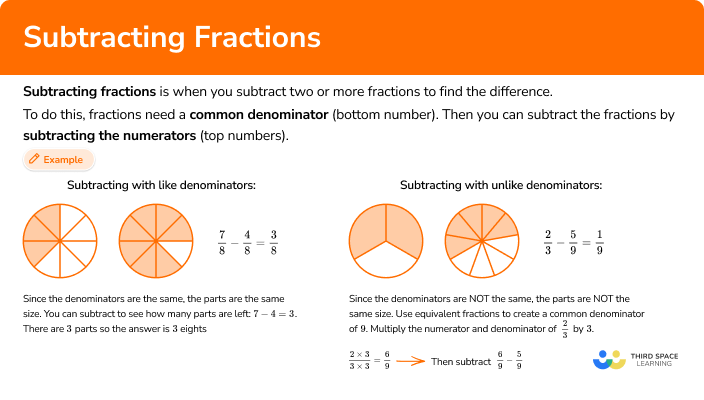## Common Core State Standards

How does this relate to 4th grade math and 5th grade math?

• Grade 4 – Number and Operations – Fractions (4.NF.B.3c)
Add and subtract mixed numbers with like denominators, e.g., by replacing each mixed number with an equivalent fraction, and/or by using properties of operations and the relationship between addition and subtraction.

• Grade 5 – Number and Operations – Fractions (5.NF.A.1)
Add and subtract fractions with unlike denominators (including mixed numbers) by replacing given fractions with equivalent fractions in such a way as to produce an equivalent sum or difference of fractions with like denominators.
For example, \cfrac{2}{3} + \cfrac{5}{4} = \cfrac{8}{12} + \cfrac{15}{12} = \cfrac{23}{12}. (In general, \cfrac{a}{b} + \cfrac{c}{d} = \cfrac{(ad\; + \; bc)}{bd}. )

## How to subtract fractions

In order to subtract fractions with like denominators:

1. Subtract the numerators (top numbers).

In order to subtract mixed numbers with like denominators:

1. Subtract the fractions, borrowing if needed.
2. Subtract the whole numbers.

In order to subtract fractions with unlike denominators:

1. Create common denominators (bottom numbers).
2. Subtract the numerators (top numbers).

In order to subtract mixed numbers with unlike denominators:

1. Create common denominators (bottom numbers).
2. Subtract the fractions, borrowing if needed.
3. Subtract the whole numbers.

## Subtracting fractions examples

### Example 1: subtracting fractions with like denominators

Solve \cfrac{3}{5}-\cfrac{1}{5}.

1. Subtract the numerators (top numbers).

The equation is taking \cfrac{1}{5} away from \cfrac{3}{5}.

Since the denominators are the same, the parts are the same size. You subtract to see how many parts are left: 3-1=2.

There are 2 parts. But what size are the parts? They are still fifths, so the denominator stays the same.

\cfrac{3}{5}-\cfrac{1}{5}=\cfrac{2}{5}

### Example 2: subtracting mixed numbers with like denominators

Solve 4 \cfrac{11}{12}-1 \cfrac{7}{12}.

The equation is taking 1 \cfrac{7}{12} away from 4 \cfrac{11}{12}.

Start with the fractions. Since the denominators are the same, the parts are the same size. You subtract to see how many parts are left: 11-7=4.

There are 4 parts. But what size are the parts? They are still twelfths, so the denominator stays the same.

\cfrac{11}{12}-\cfrac{7}{12}=\cfrac{4}{12}

4-1=3

4 \cfrac{11}{12}-1 \cfrac{7}{12}=3 \cfrac{4}{12}

You can also write this answer as the equivalent fraction 3 \cfrac{1}{3}.

### Example 3: subtracting mixed numbers with like denominators

Solve 2 \cfrac{3}{10}-1 \cfrac{9}{10}.

The equation is taking 1 \cfrac{9}{10} away from 2 \cfrac{3}{10}.

Start with the fractions. Since the denominators are the same, the parts are the same size. However, there are not enough parts to take 9 away from 3.

You can break one of the wholes into \cfrac{10}{10}

2 \cfrac{3}{10}=1 \cfrac{13}{10}

Now you can solve 1 \cfrac{13}{10}-1 \cfrac{9}{10}.

You subtract to see how many parts are left: 13-9=4.

There are 4 parts. But what size are the parts? They are still tenths, so the denominator stays the same.

\cfrac{13}{10}-\cfrac{9}{10}=\cfrac{4}{10}

1-1=0

Sometimes the answer will be just a fraction.

1 \cfrac{13}{10}-1 \cfrac{9}{10}=\cfrac{4}{10}

You can also write this answer as the equivalent fraction \cfrac{2}{5} or the decimal 0.4.

### Example 4: subtracting fractions with unlike denominators

Solve \cfrac{3}{4}-\cfrac{1}{2}.

Since \cfrac{3}{4} and \cfrac{1}{2} do not have like denominators, the parts are NOT the same size. A common denominator of 4 can be used.

Multiply the numerator and denominator of \cfrac{1}{2} by 2 to create an equivalent fraction.

\cfrac{3}{4} \quad and \quad \cfrac{1 \; \times \; 2}{2 \; \times \; 2}=\cfrac{2}{4}

Now use the equivalent fraction to solve: \cfrac{3}{4}-\cfrac{2}{4}.

Since the denominators are the same, the parts are the same size. You subtract to see how many parts are left: 3-2=1.

There is 1 part. But what size is the part? It is still a fourth, so the denominator stays the same.

\cfrac{3}{4}-\cfrac{2}{4}=\cfrac{1}{4}

### Example 5: subtracting mixed numbers with unlike denominators

Solve 2 \cfrac{1}{2}-1 \cfrac{1}{3}.

The equation is taking 1 \cfrac{1}{3} away from 2 \cfrac{1}{2}.

Start with the fractions. Since \cfrac{1}{2} and \cfrac{1}{3} do not have like denominators, the parts are NOT the same size.

Multiply the numerator and denominator by the opposite denominator to create equivalent fractions with common denominators.

\cfrac{1 \; \times \; 3}{2 \; \times \; 3}=\cfrac{3}{6} \quad and \quad \cfrac{1 \; \times \; 2}{3 \; \times \; 2}=\cfrac{2}{6}

Now use the equivalent fractions to solve: 2 \cfrac{3}{6}-1 \cfrac{2}{6}.

Start with the fractions. Since the denominators are the same, the parts are the same size. You subtract to see how many parts are left: 3-2=1.

There is 1 part. But what size is the part? It is still a sixth, so the denominator stays the same.

\cfrac{3}{6}-\cfrac{2}{6}=\cfrac{1}{6}

2-1=1

2 \cfrac{3}{6}-1 \cfrac{2}{6}=1 \cfrac{1}{6}

### Example 6: subtracting mixed numbers with unlike denominators

Solve 5 \cfrac{3}{8}-1 \cfrac{3}{4}.

The equation is taking 1 \cfrac{3}{4} away from 5 \cfrac{3}{8}.

Start with the fractions. Since \cfrac{3}{8} and \cfrac{3}{4} do not have like denominators, the parts are NOT the same size. A common denominator of 8 can be used.

Multiply the numerator and denominator of \cfrac{3}{4} by 2 to create an equivalent fraction.

\cfrac{3}{8} \quad and \quad \cfrac{3 \; \times \; 2}{4 \; \times \; 2}=\cfrac{6}{8}

Now use the equivalent fractions to solve: 5 \cfrac{3}{8}-1 \cfrac{6}{8}.

Start with the fractions. Since the denominators are the same, the parts are the same size. However, there are not enough parts to take 6 away from 3.

You can break one of the wholes into \cfrac{8}{8}…

5 \cfrac{3}{8}=4 \cfrac{11}{8}

Now you can solve 4 \cfrac{11}{8}-1 \cfrac{6}{8}.

You subtract to see how many parts are left: 11-6=5.

There are 5 parts left. But what size are the parts? They are still eighths, so the denominator stays the same.

\cfrac{11}{8}-\cfrac{6}{8}=\cfrac{5}{8}

4-1=3

4 \cfrac{11}{8}-1 \cfrac{6}{8}=3 \cfrac{5}{8}

### Teaching tips for subtracting fractions

• Fraction work in 3rd grade centers around understanding through models, particularly area models and number lines. To build on this in 4th grade and 5th grade, always have physical or digital models available for students to use when necessary.

• To introduce this topic, give students a fraction subtraction problem and give them time to solve in a way that makes sense to them. Then go over the different solving strategies as a whole group.

• In the beginning, you may want to stick to smaller fractions or problems that do not involve borrowing. However, at some point it is important to transition to showing students all kinds of fraction subtraction problems mixed together. This gives them an opportunity to identify which solving strategy to use.

• Fraction worksheets may have their place when students are still developing understanding around addition, but once students have a successful strategy and can flexibly operate, incorporate math games or real world projects that involve subtraction of fractions.

### Our favorite mistakes

• Subtracting without like denominators
When fractions are subtracted, they must have like denominators. Before subtracting fractions with unlike denominators, use equivalent fractions to create a common denominator.

• When subtracting fractions, only the numerators are subtracted
The denominator tells us how big the parts are. You subtract the number of parts (numerators) and keep the denominator (the size of the parts) the same.

### Subtracting fractions practice questions

1. Solve \cfrac{5}{6}-\cfrac{2}{6}.

\cfrac{3}{6}\cfrac{3}{0}31 \cfrac{1}{6}The equation is taking \cfrac{2}{6} away from \cfrac{5}{6}.

Since the denominators are the same, the parts are the same size. You subtract to see how many parts are left: 5-2=3.There are 3 parts. But what size are the parts? They are still sixths, so the denominator stays the same.\cfrac{5}{6}-\cfrac{2}{6}=\cfrac{3}{6}

You can also write this answer as the equivalent fraction \cfrac{1}{2}.

2. Solve 3 \cfrac{1}{4}-1 \cfrac{3}{4}.

2 \cfrac{2}{4}\cfrac{3}{4}1 \cfrac{2}{4}3The equation is taking 1 \cfrac{3}{4} away from 3 \cfrac{1}{4}.

Start with the fractions. Since the denominators are the same, the parts are the same size. However, there are not enough parts to take 3 away from 1.

You can break one of the wholes into \cfrac{4}{4}…

3 \cfrac{1}{4}=2 \cfrac{5}{4}Now you can solve 2 \cfrac{5}{4}-1 \cfrac{3}{4}.

You subtract to see how many parts are left: 5-3=2.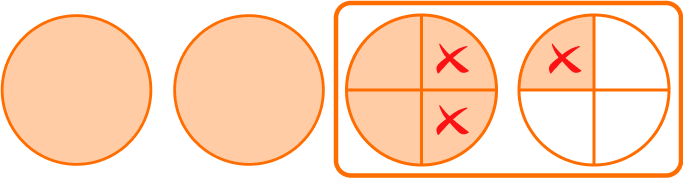There are 2 parts. But what size are the parts? They are still fourths, so the denominator stays the same.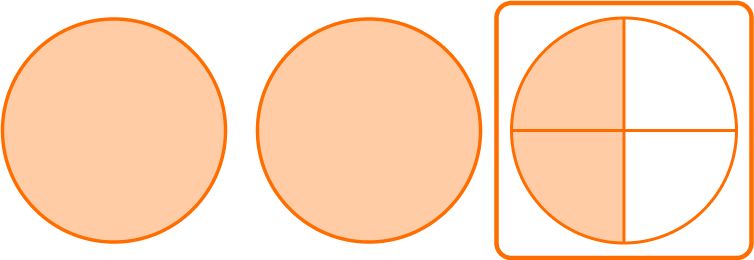\cfrac{5}{4}-\cfrac{3}{4}=\cfrac{2}{4}

Subtract the whole numbers.2-1=12 \cfrac{5}{4}-1 \cfrac{3}{4}=1 \cfrac{2}{4}

You can also write this answer as the equivalent mixed number 1 \cfrac{1}{2}.

3. Solve 4 \cfrac{8}{10}-2 \cfrac{1}{10}.

3 \cfrac{7}{10}6 \cfrac{9}{10}2 \cfrac{9}{10}2 \cfrac{7}{10}The equation is taking 2 \cfrac{1}{10} away from 4 \cfrac{8}{10}.

Start with the fractions. Since the denominators are the same, the parts are the same size. You subtract to see how many parts are left: 8-1=7.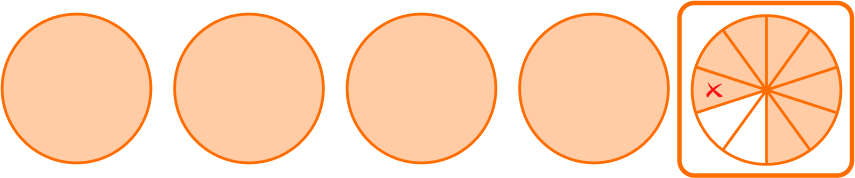There are 7 parts. But what size are the parts? They are still tenths, so the denominator stays the same.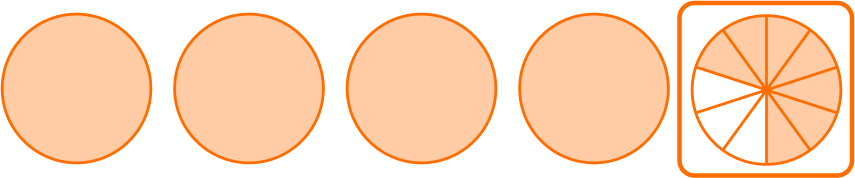\cfrac{8}{10}-\cfrac{1}{10}=\cfrac{7}{10}

Subtract the whole numbers.4-2=24 \cfrac{8}{10}-2 \cfrac{1}{10}=2 \cfrac{7}{10}

You can also write this answer as the decimal number 2.7.

4. Solve \cfrac{2}{3}-\cfrac{1}{5}.

\cfrac{1}{2}\cfrac{7}{15}\cfrac{3}{8}\cfrac{7}{0}Since \cfrac{2}{3} and \cfrac{1}{5} do not have like denominators, the parts are NOT the same size.

Multiply the numerator and denominator by the opposite denominator to create equivalent fractions with common denominators.

\cfrac{2 \; \times \; 5}{3 \; \times \; 5}=\cfrac{10}{15} \quad and \quad \cfrac{1 \; \times \; 3}{5 \; \times \; 3}=\cfrac{3}{15}

Now use the equivalent fractions to solve: \cfrac{10}{15}-\cfrac{3}{15}.

Since the denominators are the same, the parts are the same size. You subtract to see how many parts are left: 10-3=7.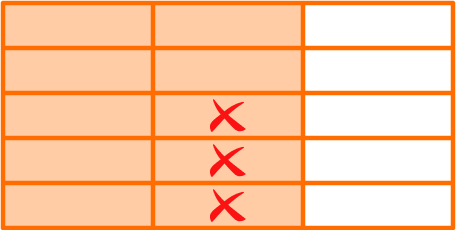There are 7 parts. But what size are the parts? They are still fifteenths, so the denominator stays the same.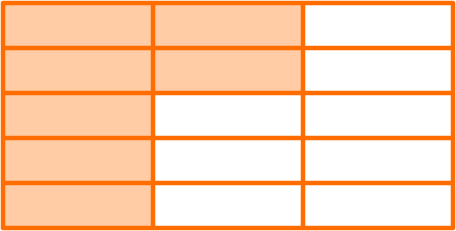\cfrac{10}{15}-\cfrac{3}{15}=\cfrac{7}{15}

5. Solve 3\cfrac{3}{4}-2\cfrac{1}{3}.

4\cfrac{1}{12}1\cfrac{5}{12}2\cfrac{5}{12}1\cfrac{7}{12}The equation is taking 2\cfrac{1}{3} away from 3\cfrac{3}{4}.

Start with the fractions. Since \cfrac{3}{4} and \cfrac{1}{3} do not have like denominators, the parts are NOT the same size.

Multiply the numerator and denominator by the opposite denominator to create equivalent fractions with common denominators.

\cfrac{3 \; \times \; 3}{4 \; \times \; 3}=\cfrac{9}{12} \quad and \quad \cfrac{1 \; \times \; 4}{3 \; \times \; 4}=\cfrac{4}{12}

Now use the equivalent fractions to solve: 3 \cfrac{9}{12}-2 \cfrac{4}{12}.

Start with the fractions. Since the denominators are the same, the parts are the same size. You subtract to see how many parts are left: 9-4=5.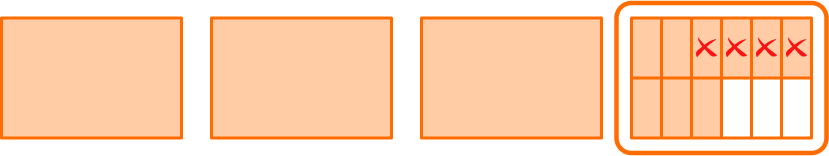There are 5 parts. But what size are the parts? They are still twelfths, so the denominator stays the same.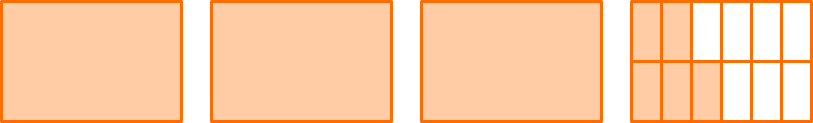\cfrac{9}{12}-\cfrac{4}{12}=\cfrac{5}{12}

Subtract the whole numbers.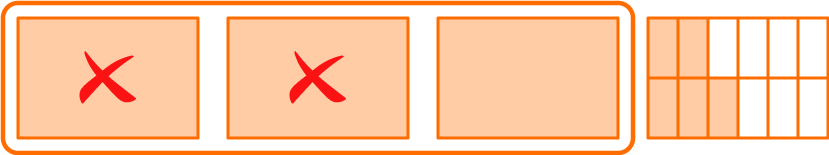3-2=1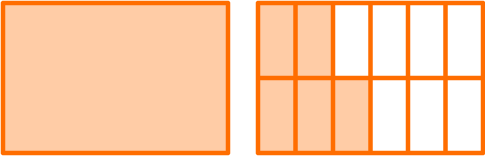3 \cfrac{9}{12}-2 \cfrac{4}{12}=1 \cfrac{5}{12}

6. Solve 5 \cfrac{1}{12}-3 \cfrac{5}{6}.

4\cfrac{1}{12}1\cfrac{3}{12}2\cfrac{5}{12}1\cfrac{7}{12}The equation is taking 3 \cfrac{5}{6} away from 5 \cfrac{1}{12}.

Start with the fractions. Since \cfrac{1}{12} and \cfrac{5}{6} do not have like denominators, the parts are NOT the same size. A common denominator of 12 can be used.

Multiply the numerator and denominator of \cfrac{5}{6} by 2 to create an equivalent fraction.

\cfrac{1}{12} \quad and \quad \cfrac{5 \; \times \; 2}{6 \; \times \; 2}=\cfrac{10}{12}

Now use the equivalent fraction to solve: 5 \cfrac{1}{12}-3 \cfrac{10}{12}.

Start with the fractions. Since the denominators are the same, the parts are the same size. However, there are not enough parts to take 10 away from 1.

You can break one of the wholes into \cfrac{12}{12}…

5 \cfrac{1}{12}=4 \cfrac{13}{12}Now you can solve 4 \cfrac{13}{12}-3 \cfrac{10}{12}.

You subtract to see how many parts are left: 13-10=3.There are 3 parts left. But what size are the parts? They are still twelfths, so the denominator stays the same.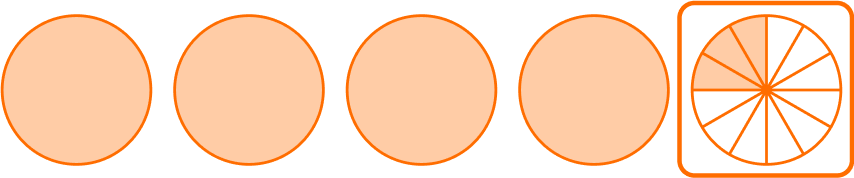\cfrac{13}{12}-\cfrac{10}{12}=\cfrac{3}{12}

Subtract the whole numbers.4-3=14 \cfrac{13}{12}-3 \cfrac{10}{12}=1 \cfrac{3}{12}

You can also write this answer as the equivalent mixed number 1 \cfrac{1}{4}.

Does multiplying by a common factor change the size of the fraction?

No, although a new numerator and new denominator are created the value of the fraction remains the same. Since the new fraction has a larger denominator, the parts will be smaller, so the numerator also needs to be larger so that the total of the parts is the same size as the original fraction.

Do all fraction differences need to be in lowest terms?

No, students do not have to find the least common denominator in order to correctly answer a fraction addition question. However, as students progress in their understanding of fractions, it is a good habit to grow. It is also important to be mindful of standard expectations, as they may vary from state to state.

Is addition of fractions similar to subtraction of fractions?

Yes, you follow many of the same steps to add fractions, which also requires common denominators. The only difference is that you add the numerators instead of subtracting.

## Still stuck?

At Third Space Learning, we specialize in helping teachers and school leaders to provide personalized math support for more of their students through high-quality, online one-on-one math tutoring delivered by subject experts.

Each week, our tutors support thousands of students who are at risk of not meeting their grade-level expectations, and help accelerate their progress and boost their confidence.